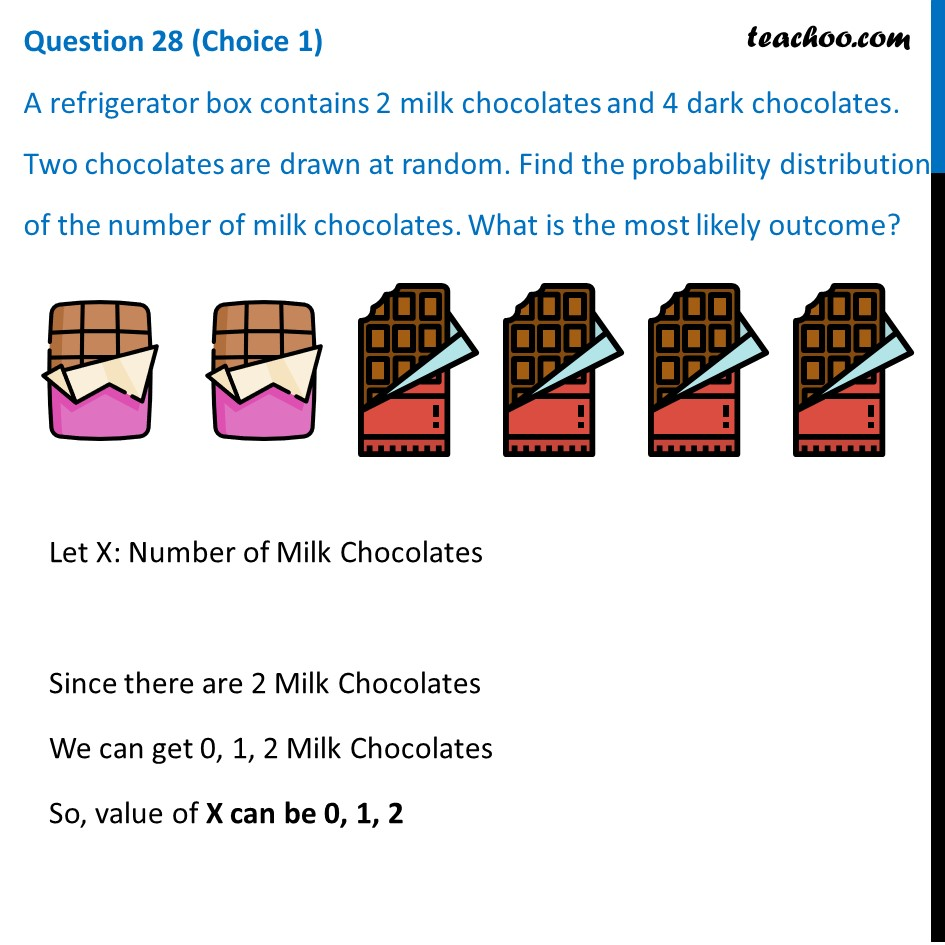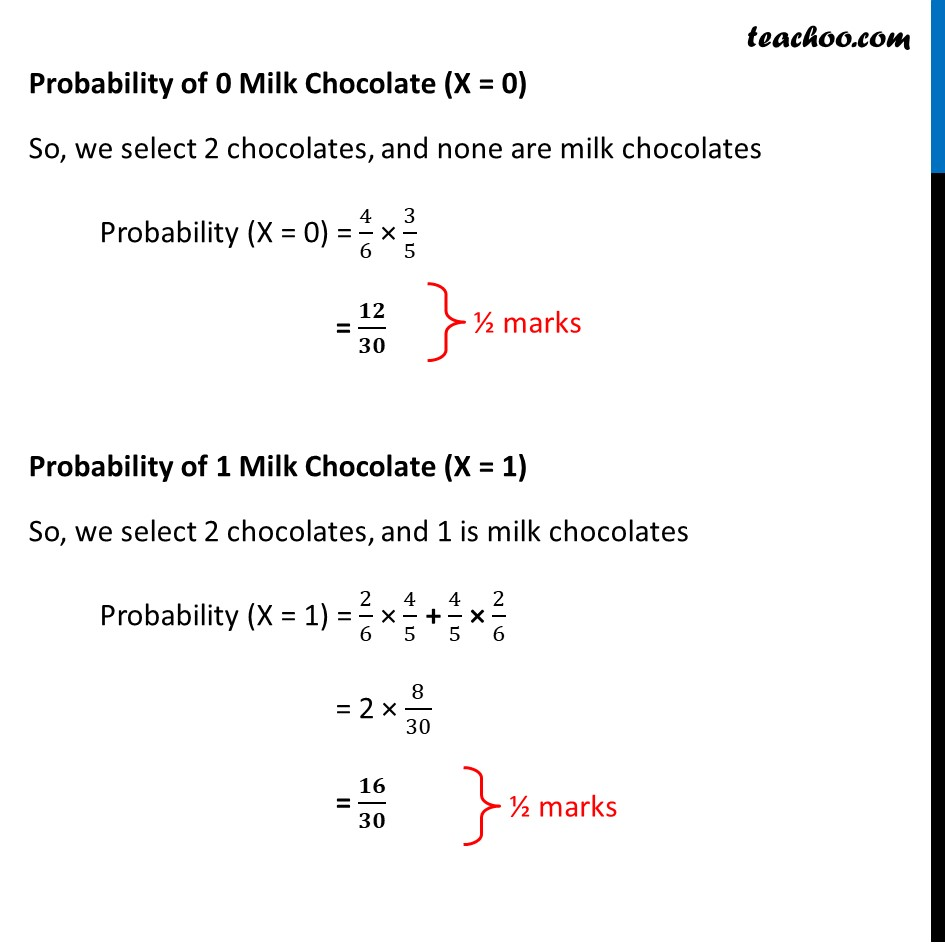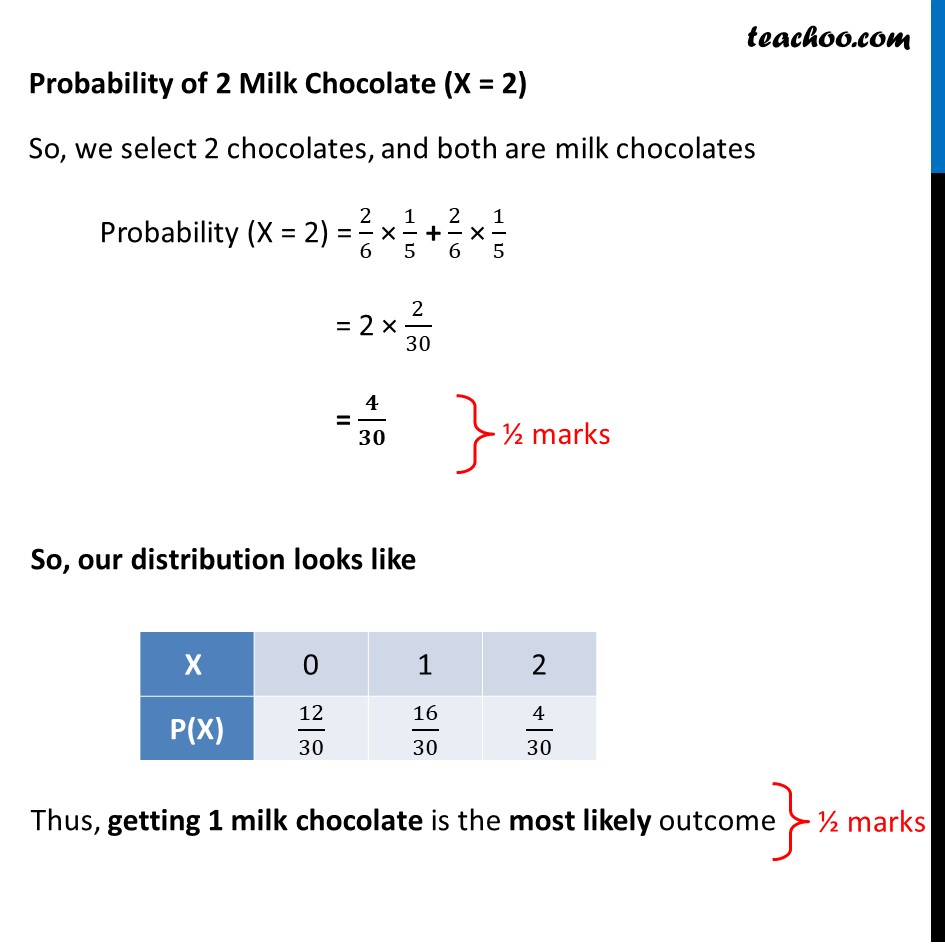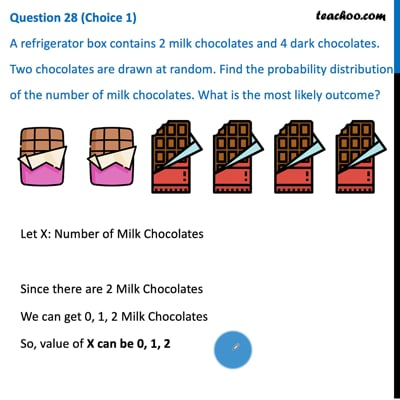CBSE Class 12 Sample Paper for 2021 Boards

Class 12
Solutions of Sample Papers and Past Year Papers - for Class 12 Boards

## A refrigerator box contains 2 milk chocolates and 4 dark chocolates. Two chocolates are drawn at random. Find the probability distribution of the number of milk chocolates. What is the most likely outcome?This video is only available for Teachoo black users

Introducing your new favourite teacher - Teachoo Black, at only ₹83 per month

### Transcript

Question 28 (Choice 1) A refrigerator box contains 2 milk chocolates and 4 dark chocolates. Two chocolates are drawn at random. Find the probability distribution of the number of milk chocolates. What is the most likely outcome? Let X: Number of Milk Chocolates Since there are 2 Milk Chocolates We can get 0, 1, 2 Milk Chocolates So, value of X can be 0, 1, 2 Probability of 0 Milk Chocolate (X = 0) So, we select 2 chocolates, and none are milk chocolates Probability (X = 0) = 4/6 × 3/5 = 𝟏𝟐/𝟑𝟎 Probability of 1 Milk Chocolate (X = 1) So, we select 2 chocolates, and 1 is milk chocolates Probability (X = 1) = 2/6 × 4/5 + 4/5 × 2/6 = 2 × 8/30 = 𝟏𝟔/𝟑𝟎 Probability of 2 Milk Chocolate (X = 2) So, we select 2 chocolates, and both are milk chocolates Probability (X = 2) = 2/6 × 1/5 + 2/6 × 1/5 = 2 × 2/30 = 𝟒/𝟑𝟎 So, our distribution looks like Thus, getting 1 milk chocolate is the most likely outcome